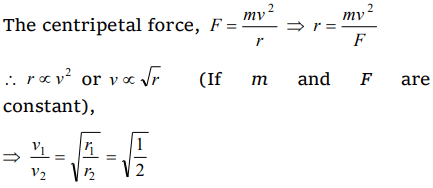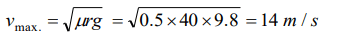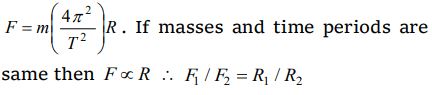## Motion in a Plane Questions and Answers Part-11

1. A person with his hands in his pockets is skatingon ice at the velocity of 10 m/s and describes a circle of radius 50 m. What is his inclination with vertical
a) $\tan^{-1}\left(\frac{1}{10}\right)$
b) $\tan^{-1}\left(\frac{3}{5}\right)$
c) $\tan^{-1}\left(1\right)$
d) $\tan^{-1}\left(\frac{1}{5}\right)$

Explanation:2. If the radius of curvature of the path of two particles of same masses are in the ratio 1 : 2, then in order to have constant centripetal force, their velocity, should be in the ratio of
a) 1 : 4
b) 4 : 1
c) $\sqrt{2}:1$
d) $1:\sqrt{2}$

Explanation:3. An object is moving in a circle of radius 100 m with a constant speed of 31.4 m/s. What is its average speed for one complete revolution
a) Zero
b) 31.4 m/s
c) 3.14 m/s
d) $\sqrt{2}\times 4. 31m/s$

Explanation: As the speed is constant throughout the circular motion therefore its average speed is equal to instantaneous speed

4. A body of mass 1 kg tied to one end of string is revolved in a horizontal circle of radius 0.1 m with a speed of 3 revolution/sec, assuming the effect of gravity is negligible, then linear velocity, acceleration and tension in the string will be?
a) $1.88 m \diagup s,35.5 m \diagup s^{2} , 35.5 N$
b) $2.88 m \diagup s,45.5 m \diagup s^{2} , 45.5 N$
c) $3.88 m \diagup s,55.5 m \diagup s^{2} , 55.5 N$
d) None of these

Explanation:Tension in string,
T = $m \omega^{2} r = 1\times(6\pi)^{2}\times0.1 = 35.5 N$

5. The acceleration of a train travelling with speed of 400 m/s as it goes round a curve of radius 160 m, is
a) $1 km\diagup s^{2}$
b) $100 m\diagup s^{2}$
c) $10 m\diagup s^{2}$
d) $1 m\diagup s^{2}$

Explanation:6. A car of mass 800 kg moves on a circular track of radius 40 m. If the coefficient of friction is 0.5, then maximum velocity with which the car can move is
a) 7 m/s
b) 14 m/s
c) 8 m/s
d) 12 m/s

Explanation:7. A 500 kg crane takes a turn of radius 50 m with velocity of 36 km/hr. The centripetal force is
a) 1200 N
b) 1000 N
c) 750 N
d) 250 N

Explanation:8. Two bodies of equal masses revolve in circular orbits of radii $R_{1}$ and $R_{2}$ with the same period. Their centripetal forces are in the ratio
a) $\left(\frac{R_{2}}{R_{1}}\right)^{2}$
b) $\frac{R_{1}}{R_{2}}$
c) $\left(\frac{R_{1}}{R_{2}}\right)^{2}$
d) $\sqrt{R_{1}R_{2}}$

Explanation:9. In case of uniform circular motion which of the following physical quantity do not remain constant
a) Speed
b) Momentum
c) Kinetic energy
d) Mass

10. What happens to the centripetal acceleration of a revolving body if you double the orbital speed v and half the angular velocity $\omega$